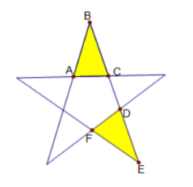$\text{A.}$ $60^{\circ}$ $\text{B.}$ $120^{\circ}$ $\text{C.}$ $72^{\circ}$ $\text{D.}$ $144^{\circ}$
【答案】 D